Prep for Calculus Flashcards Preview

Math and Calculus > Prep for Calculus > Flashcards

Flashcards in Prep for Calculus Deck (52):
1

2

3

4

5

6

7

8

9

10

11

12

13

14

15

16

17

18

19

20

f(x)=x321

f(x)=x^(1/2)22

f(x)=|x|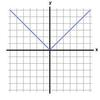23

Rational function f(x)=(1/x)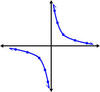24

sine function f(x)=sin x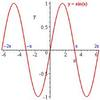25

cosine function f(x)=cos x26

Transformations of functions f(x)=x2 f(x)=x2+C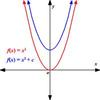27

Transformations of functions   f(x)=x2  f(x)=(x+1)228

Transformations of Functions f(x)=x2 f(x)=-x2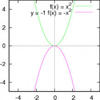29

Transformations of functions   f(x)=x2 f(x)=(x-2)2+4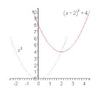30

31

32

33

34

35

36

37

38

39

40

41

42

43

44

45

46

47

48

49
50

51

52